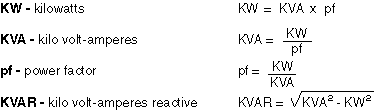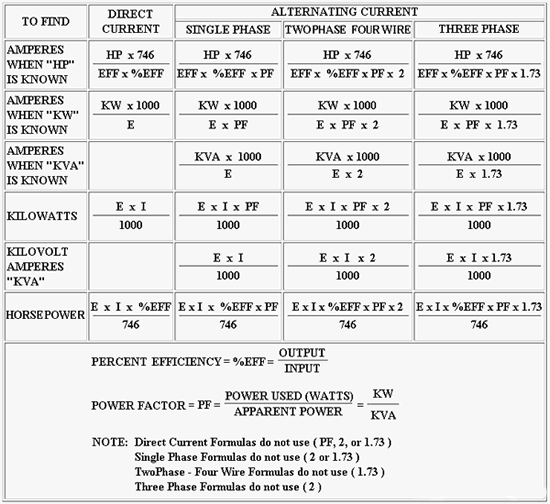### Useful Calculations and Formulas###### Three Phase Values

• For 208 volts x 1.732, use 360
• For 230 volts x 1.732, use 398
• For 240 volts x 1.732, use 416
• For 440 volts x 1.732, use 762
• For 460 volts x 1.732, use 797
• For 480 Volts x 1.732, use 831

E = Voltage / I = Amps /W = Watts / PF = Power Factor / Eff = Efficiency / HP = Horsepower